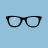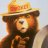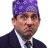# Convert 3D Oscillator from MT4

F

#### Frozenfox

##### New member
Hello everyone,

Ive been visiting the forum for a few months and it is a great source of information. Id just like to say thank you to all those who have the knowledge and contribute here on a regular basis.

I'm new to trading and am in the process of putting a Forex trading strategy together, this strategy will be based on using indicators to trade the daily charts.
I'm aware the majority of Forex traders use Meta Trader and Trading View, but i'm comfortable with Think or swim and would like to stay on the platform. After extensive research i've found numerous indicators (some developed specifically for forex) that i would like to test in my setup. IT appears after searching that, as i am aware, none are coded for Think or Swim. My ultimate goal is to be able to convert the code myself but this is something i need to develop over time.

If anyone is willing and has the time i would be most grateful if you would be willing to convert some of these codes for me? This is an oscillator that seems highly recommended for entry signals. I don't want to take advantage of anyone's generosity here i simply do no know how to go about such a code conversion.

Code:
``````//+------------------------------------------------------------------+
//|                                                 3D Oscilator.mq4 |
//|                                                                  |
//|                                                                  |
//+------------------------------------------------------------------+
#property copyright "Author - Luis Damiani. Ramdass - Conversion only"

#property indicator_separate_window
#property indicator_buffers 4
#property indicator_color1 Magenta
#property indicator_color2 DarkSlateBlue
#property indicator_color3 GreenYellow
#property indicator_color4 Red
//---- input parameters

extern int D1RSIPer=13;
extern int D2StochPer=8;
extern int D3tunnelPer=8;
extern double hot=0.4;
extern int sigsmooth=4;

//---- buffers
double sig1n[];
double sig2n[];
double upX[],dnX[];

//+------------------------------------------------------------------+
//| Custom indicator initialization function                         |
//+------------------------------------------------------------------+
int init()
{
string short_name;
//---- indicator line
SetIndexStyle(0,DRAW_LINE);
SetIndexStyle(1,DRAW_LINE);
SetIndexBuffer(0,sig1n);
SetIndexBuffer(1,sig2n);

SetIndexStyle(2,DRAW_ARROW);
SetIndexArrow(2,159);
SetIndexBuffer(2,upX);
SetIndexStyle(3,DRAW_ARROW);
SetIndexArrow(3,159);
SetIndexBuffer(3,dnX);
//----
SetIndexDrawBegin(0,D1RSIPer+D2StochPer+D3tunnelPer+hot+sigsmooth);
SetIndexDrawBegin(1,D1RSIPer+D2StochPer+D3tunnelPer+hot+sigsmooth);
SetIndexDrawBegin(2,D1RSIPer+D2StochPer+D3tunnelPer+hot+sigsmooth);
SetIndexDrawBegin(3,D1RSIPer+D2StochPer+D3tunnelPer+hot+sigsmooth);

//----
return(0);
}
//+------------------------------------------------------------------+
//| CCI_Woodies                                                         |
//+------------------------------------------------------------------+
int start()
{
int i,i2,counted_bars=IndicatorCounted();
double rsi,maxrsi,minrsi,storsi,E3D,
sig1,sig2,sk,ss,sk2;
double cs;
bool init=true;

//----
cs= D1RSIPer+D2StochPer+D3tunnelPer+hot+sigsmooth;
if(Bars<=cs) return(0);

//if (init)
//{
ss=sigsmooth;
if (ss<2) ss=2;
sk = 2 / (ss + 1);
sk2=2/(ss*0.8+1);
init=false;
//};

//---- initial zero
if(counted_bars<1)
{
for(i=1;i<=cs;i++) sig1n[Bars-i]=0.0;
for(i=1;i<=cs;i++) sig2n[Bars-i]=0.0;
for(i=1;i<=cs;i++) upX[Bars-i]=0.0;
for(i=1;i<=cs;i++) dnX[Bars-i]=0.0;
}

i=Bars-cs-1;

if (counted_bars>=cs) i=300; //i=Bars-counted_bars-1;
while (i>=0)
{

rsi=iRSI(NULL,0,D1RSIPer,PRICE_CLOSE,i);
maxrsi=rsi;
minrsi=rsi;

for (i2=i+D2StochPer;i2>=i; i2--)
{
rsi=iRSI(NULL,0,D1RSIPer,PRICE_CLOSE,i2);
if (rsi>maxrsi) maxrsi=rsi;
if (rsi<minrsi) minrsi=rsi;
//maxrsi=Maximum(rsi,maxrsi);
//minrsi=min(rsi,minrsi);
}

storsi=((rsi-minrsi)/(maxrsi-minrsi)*200-100);
E3D=hot*iCCI(NULL,0,D3tunnelPer,PRICE_TYPICAL,i)+(1-hot)*storsi;
sig1n[i]=sk*E3D+(1-sk)*sig1;
sig2n[i]=sk2*sig1+(1-sk2)*sig2;
sig1=sig1n[i];
sig2=sig2n[i];

if (sig1n[i]>sig2n[i] && sig1n[i+1]<sig2n[i+1]) upX[i]=sig2n[i]-15;
if (sig1n[i]<sig2n[i] && sig1n[i+1]>sig2n[i+1]) dnX[i]=sig2n[i]+15;

i--;
}

return(0);
}
//+------------------------------------------------------------------+``````Convert TrendFlex Oscillator - John Ehlers to thinkorswim? Questions 12How do I convert this ATR% chart study into a scanner watchlist column? Questions 5Convert encoded Mt4 to Thinkscript Questions 2